Wei, X., Sun, L., Sun, Q., Xu, S., Zhou, H., and Du, C. (2019). "Propagation velocity model of stress wave in longitudinal section of tree in different angular directions," BioRes. 14(4), 8904-8922.

#### Abstract

In order to detect the size and shape of defects inside wood, a propagation velocity model of stress wave in the longitudinal section of trees in different direction angles was proposed and evaluated. The propagation velocity model was established through theoretical analysis. Four representative tree species in the northeast region of China were taken as test samples. The propagation velocity of stress wave in the longitudinal section of trees in different directions was measured using a nondestructive testing instrument. The corresponding regression model was obtained, which was in good agreement with the theoretical mathematical model. For the larch log samples, a healthy multiple regression model (z = 109.2×2 – 182.1y2 + 36.78x2y2 – 34.76x2y4 + 1627) with correlation coefficient R2 = 0.97 and root mean square error RMSE =17.81 was used to conduct two-dimensional imaging of defective logs. Based on the results of two-dimensional imaging, the highest fitness of the images was 94.24%, and the lowest error rates of defect cavities was 6.11%. The imaging results showed that this method accurately detected the internal defects of trees and was not affected by the size of defects.

Propagation Velocity Model of Stress Wave in Longitudinal Section of Tree in Different Angular Directions

Xiwen Wei,a,b Liping Sun,a,* Quansheng Sun,a,* Shuzheng Xu,a Hongjv Zhou,a and Chunxiao Du c

In order to detect the size and shape of defects inside wood, a propagation velocity model of stress wave in the longitudinal section of trees in different direction angles was proposed and evaluated. The propagation velocity model was established through theoretical analysis. Four representative tree species in the northeast region of China were taken as test samples. The propagation velocity of stress wave in the longitudinal section of trees in different directions was measured using a nondestructive testing instrument. The corresponding regression model was obtained, which was in good agreement with the theoretical mathematical model. For the larch log samples, a healthy multiple regression model (z = 109.2x2 – 182.1y2 + 36.78x2y2 – 34.76x2y4 + 1627) with correlation coefficient R= 0.97 and root mean square error RMSE =17.81 was used to conduct two-dimensional imaging of defective logs. Based on the results of two-dimensional imaging, the highest fitness of the images was 94.24%, and the lowest error rates of defect cavities was 6.11%. The imaging results showed that this method accurately detected the internal defects of trees and was not affected by the size of defects.

Keywords: Stress wave nondestructive testing; Trees; Direction angle; Longitudinal Section;Two-dimensional imaging

Contact information: a: College of Mechanical and Electrical Engineering, Northeast Forestry University, Harbin, 150040, China; b: Department of electrical and Information Engineering, Heilongjiang University of Technology, Jixi, 158100, China; c: Harbin Veterinary Research Institute, Chinese Academy of Agricultural Sciences, Harbin, 150040, China; *Corresponding author: zdhslp@163.com; hrbsqs@126.com

INTRODUCTION

Internal defects such as decay and voids affect the physical and mechanical properties of wood, reduce the quality of wood, and affect its value. Therefore, rapid and accurate detection of wood to ascertain decay and internal defects improves the utilization rate of wood (Bucur 2005; Xu et al. 2018; Simic et al. 2019). Nondestructive testing technology does not destroy the properties and surface stability of testing samples. The application of non-destructive testing technology in wood detection has been well developed (Wang et al. 2001; Watanabe et al. 2008).

Stress wave detection technology can detect the mechanical properties and internal defects of wood. It is the most applicable and widely used non-destructive testing technology for wood (Yang and Wang 2005). The basic principle is to use a pulse hammer to strike the transmitter sensor on the tested wood, so that the mechanical stress wave is generated inside the wood under stress. The propagation time of the stress wave from the transmitting end to the receiving end is measured and translated into the propagation velocity in the corresponding direction. The physical and mechanical properties and internal defects of wood are determined by analyzing the variation of stress wave propagation time and velocity (Liao et al. 2017). Stress wave imaging technology is used to analyze and understand the propagation law of stress wave in wood, collect the original propagation time and velocity data, and use the appropriate mathematical function model or spatial interpolation method to reconstruct the internal conditions by image (Feng et al. 2014; Du et al. 2015, 2018a). By using image processing technology to detect the location, size, and decay defects in wood, the internal situation of wood section can be visually reflected (Lin et al. 2011; Liang and Fu 2014; Du et al. 2018b). Compared with other wood non-destructive testing technologies, stress wave detection has strong anti-interference ability, long transmission distance, and no need for use of a coupling agent. The testing equipment is convenient to carry and harmless to human beings. It can detect defects of arbitrary volume and shape. There are many current reports on the non-destructive testing of wood by stress wave methods (Ross et al. 1998; Rabe et al. 2004; Liang and Fu 2012; Chang et al. 2016; Yue et al. 2019).

This paper explores the propagation law of stress waves in different angular directions and longitudinal section angles of healthy trees as the foundation of the physical and mechanical properties of wood. A theoretical model of stress wave propagation velocity was obtained for the longitudinal section of trees in different angles, and the accuracy of the theoretical model was verified through experiments. Based on the theoretical calculation and experimental verification of the larch (Larix gmelinii) log multiple regression model for fault imaging, high-precision two-dimensional images of different defect sizes were established. The defect areas in the two-dimensional space of the larch logs were clearly defined to accurately reflect the internal defects of the wood. This paper provides a new theory for quantitative characterization of internal defect decay and tree internal defects of larch vigorous wood.

Theory of Stress Wave Propagation in Longitudinal Section of Tree in Different Direction Angles

Wood is an orthogonal material with mechanical and thermal properties that are single-valued and independent in the direction of three mutually perpendicular principal axes (Romano et al. 2008). For the sake of simplicity, assuming that an ideal wood piece is a cylinder, a three-dimensional coordinate system of wood with O as the origin is established as shown in Fig. 1(a), where OL represents the longitudinal axis and is parallel to the grain direction; OR represents the radial axis, perpendicular to the growth ring; and OT represents the tangent axis, tangent to the growth ring. It is further assumed that the stress wave propagates in the OS direction in the radial axis section, O represents the position of the stress wave emitting end sensor, S represents the position of the stress wave receiving end sensor, and represents the angle between the OR and the OSi.e., the angle between the radial direction and the propagation direction of the stress wave. In addition, its cross section is considered as a regular circle. As shown in Fig. 1 (b), O represents the position of the sensor at the transmitter of stress wave, Rand R2 represent the position of the sensor at the receiver of stress wave. The stress wave propagates in the direction of OR1 and OR2. The angle between them is expressed by . The propagation velocity in the ORdirection is the radial propagation velocity, and the propagation velocity in the other directions is called the chord propagation velocity. Generally, the tensile strength in the longitudinal direction in healthy trees is higher, and the tensile strength in the radial and tangential directions is lower. The radial propagation velocity is the fastest, and the propagation velocity becomes slower as the chord angle increases (Wang et al. 2011; Liu and Li 2018).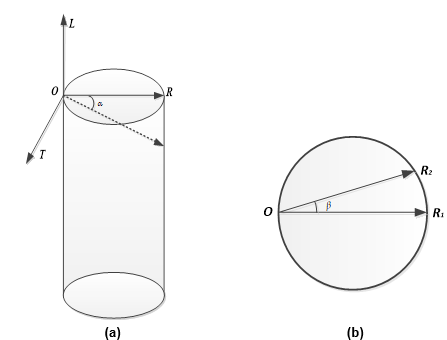Fig. 1. Schematic diagram of (a) three-dimensional coordinate system of wood, (b) stress wave propagation in cross section

In practical applications, the force direction of the wood may be different from the direction of the wood grain formed naturally. In this case, the bearing capacity of the wood twill should to be considered. According to the twill compressive strength formula of wood summarized through a large number of experiments (Mascia et al. 2011), the formula for the propagation velocity of the stress wave on the direction angle can be derived as follows,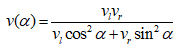(1)

where vl represents the propagation velocity of stress wave in longitudinal section, vr represents the propagation velocity of stress wave in radial axial.

If there is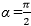, then there is v(α) = vl,; if α = 0, then there is v(α) = vr. The second-order Taylor expansion formula (Eq. 1) is used at α = 0, and the remainder is removed to obtain the following formula,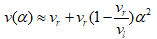(2)

where vl and vr are determined by the nature of wood itself, so the relationship between the propagation velocity of stress wave and the direction angle is approximately an open parabola. Equation 2 is used as a theoretical model for the propagation of stress waves in healthy trees in different directions.

The acoustic anisotropy of the wet material was analyzed experimentally according to Weng et al. (2016). The formula of the propagation velocity of the stress wave at the chord angle is obtained as follows,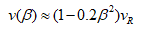(3)

where the radial velocity when the angle of the section is 0 is expressed by vR.

Equation 3 is used as a theoretical model for the propagation of stress waves in the angles of different longitudinal sections in healthy trees, which approximates a parabola with an opening downward.

By substituting Eq. 3 into Eq. 2, the propagation velocity v(α, β) of stress wave in longitudinal section with different directions is obtained as follows,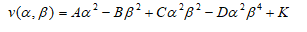(4)

where,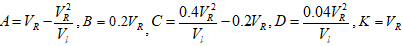.

Equation 4 is a comprehensive theoretical mathematical model of stress wave propagation velocity and direction angle and section angle in healthy trees, it can be seen that the propagation velocity model of stress wave in longitudinal section with different directions is the sum of polynomials of direction angle and section angle.

EXPERIMENTAL

Materials and Equipment

The internal structure of trees is complex, and it is divided into radial, tangential and longitudinal directions. The propagation velocity of stress waves detected at different positions is very different (Huan et al. 2018). Therefore, tree samples were selected from representative non-node, non-decay, non-cracking, straight trunk of birch (Betula platyphylla), ashtree (Fraxinus mandshurica), elm (Ulmus pumila), and larch (Larix gmelinii) logs in Northeast China as test objects in order to avoid test errors. To ensure the theory that stress waves propagate on the vertical section of logs, the numbers of birch, ashtree, elm, and larch logs were counted as Y1, Y2, Y3, and Y4, respectively. The diameter at breast height (DBH) and height of healthy log samples were measured by a leather tape measure and a caliper. The specific basic conditions are shown in Table 1.

Table 1. Sample Basic Information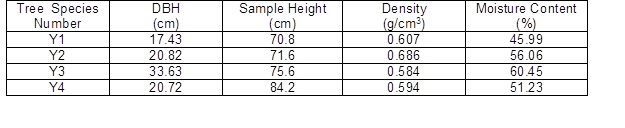The Arbotom nondestructive stress wave tester (RINNTECH, Heidelberg, Germany) was used, as shown in Fig. 2. The tester contains 12 sensors and analysis software system, which can measure the propagation time and propagation velocity of stress wave among sensors, generating the corresponding matrix reports. A 202-0 desktop dryer (YONG GUANG MING, Beijing, China) and Sanfeng electronic scale (INESA, Shanghai, China) were used to measure the moisture content of wood.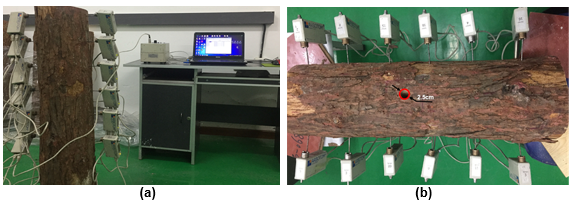Fig. 2. Setup of nondestructive testing experiments of (a) healthy sample detection, (b) defective sample detection

Methods

Measurement of stress wave propagation velocity

(1) Measurement of stress wave propagation velocity in different direction angles. A longitudinal section of the test sample was selected, and sensors were placed 5cm away from the top of the sample, 6 sensors on each side. The distance between two adjacent sensors on the same side is 10 cm, and the direction angle between the contralateral sensors was represented by α. The sensor No.1- No.6 was the transmitter, and No. 7 – No. 12 were the receiver, as shown in Fig. 3(a). The sensors of No.1- No.6 were struck in sequence, and each sensor is tapped 5 to 8 times. The propagation velocity and time of stress wave in healthy log samples were obtained by Arbotom stress wave tester. The same longitudinal section was measured 3 times and averaged.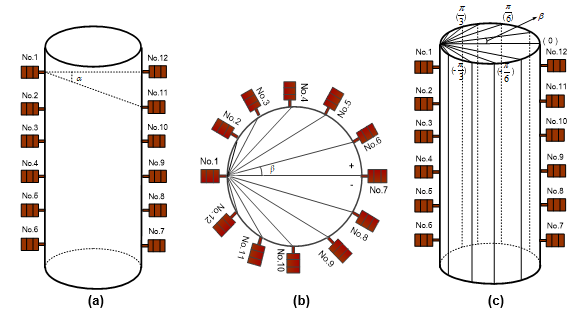Fig. 3. Distribution of stress wave sensors in (a) different direction angles, (b) different longitudinal section angles, (c) longitudinal section with different directions

(2) Measurement of stress wave propagation velocity in different longitudinal section angles. A cross section was selected with different longitudinal sections of the test sample and place sensor No. 1 1m above the ground and sensor No. 12 at the other end of the diameter at the same height, then, sensors were arranged at intervals of in positive and negative directions, as shown in Fig. 3(b). The sensor No.1 was the transmitter, and No. 2 – No. 12 were the receiver, the angle between adjacent sensors was denoted by β.

(3) Measurement of stress wave propagation velocity in longitudinal section with different directions. The larch logs were selected as test samples, and the sensor arrangement of the stress wave tester was shown in Fig. 3(c). Sensors No.1 – No.6 were used as the transmitting end, and No.7 – No.12 were the receiving end. The samples propagation velocities of the 11 longitudinal section stress wave in different directions were measured.

(4) Measurement of stress wave propagation velocity in defective logs. Circular hollow defects of 2.5 cm, 5 cm, 7.5 cm, and 10 cm in diameter were manually cut in the middle position of the larch logs, and the same experimental method was adopted for testing.

Imaging of internal defects in logs

Based on the established regression model of the stress wave propagation velocity and longitudinal section in different directions, the defect area was obtained by reconstruction of larch defect two-dimensional image (Huang et al. 2013; Liu et al. 2019). The steps are shown in Fig. 4.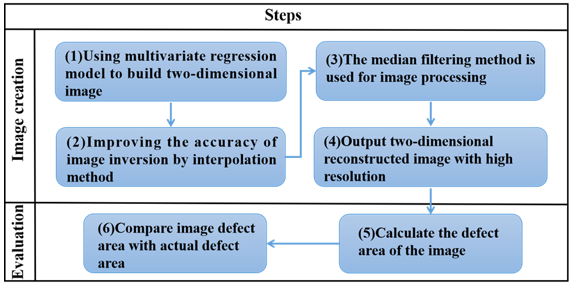Fig. 4. Steps to internal defect imaging

Determination of moisture content

The variation of wood moisture content can have a great influence on the propagation velocity of stress wave (Baranski 2018; Gao et al. 2018). In order to reduce the influence of the change of sample moisture content, the sample was packaged during transportation, and it was transported back to the laboratory at an ambient temperature of 23 °C and air relative humidity of 54% for 48 h. The moisture content of the samples was measured after transportation. Reference was made to ISO 3130 (1975), a representative moisture content test piece with a thickness of 0.01 m was sawed at a distance of 0.24 m from the end of the sample, and the weight was regarded as the quality of the wet material of the log, which it was tested. The sample was then dried in a constant temperature oven at 103 ± 2 °C for about 24 h. The weight was taken out and recorded, and then returned to the oven for further drying. It is weighed every 4 h until the weight of the last two weights is constant, which is recorded as the absolute dry weight of the test piece, as illustrated in Fig. 5.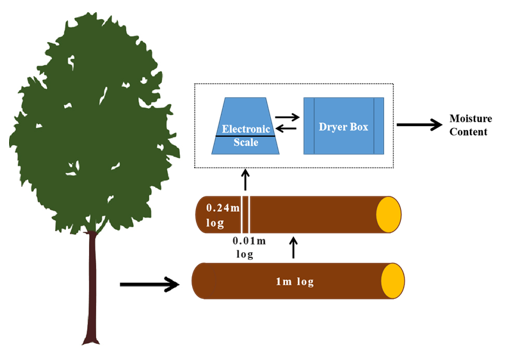Fig. 5. Moisture content testing process

The formulas for calculating the moisture content of four samples are as follows: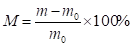(5)

where M is the moisture content of the sample(%), m is the quality of the test piece(g), and mis the quality of the test piece when it is completely dry(g).

RESULTS AND DISCUSSION

An Arbotom stress wave nondestructive wood tester was used to measure the propagation velocity of stress wave in different direction angles, longitudinal section angle of Y1-Y4 logs. The relationship between the propagation velocity and the direction angles and the longitudinal section angles was obtained.

Analysis of the Propagation Velocity of Stress Wave in Different Direction Angles

When the longitudinal section angle was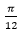, the propagation velocity of stress wave of different direction angles between the sensor No.1 and the sensor No.7- No.12 were measured on the healthy Y1-Y4 logs. The results are shown in Table 2.

The stress wave propagation velocities of the four healthy log samples in different direction angles are different. The higher the wood density was, the higher the propagation velocity was, but the variation law increased with the increase of the direction angles, and the propagation velocity in the horizontal direction was the smallest (Table 2). Using SPSS data analysis software, the data in Table 2 were plotted as scatter plots of direction angle and stress wave propagation velocity, and the curve was fitted according to the mathematical model (y = ax2 + b) of Eq. 2, as shown in Fig. 6, where the abscissa x represents direction angle α, expressed in radians, and the longitudinal coordinate y represents the stress wave propagation velocity v. The results show that the coefficients of determination R2 of the four samples were all above 0.83, and the significance P was less than 0.01, which indicates that the theoretical mathematical model of Eq. 2 had a good goodness of fit and was suitable for establishing the relationship between stress wave propagation velocity and direction angle of different tree species, which verified the correctness of the theoretical model.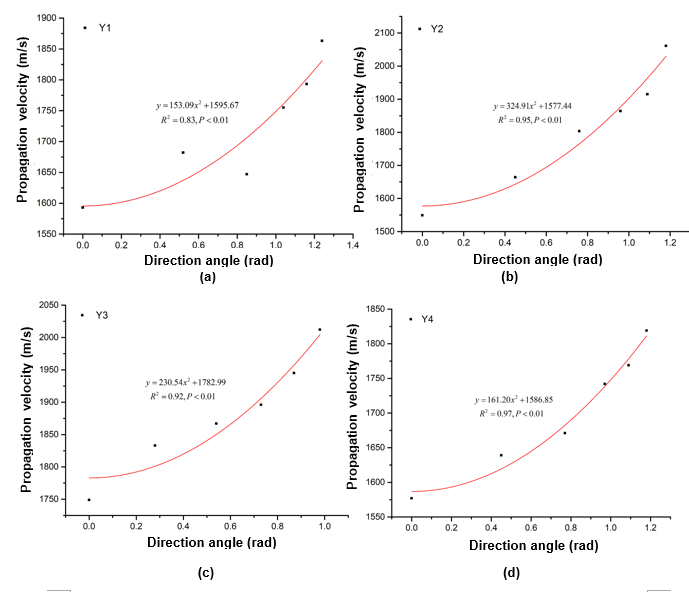Fig. 6. Fitting curve of stress wave propagation velocity in different direction angles of healthy Y1-Y4 logs

Table 2. Stress Wave Propagation Velocities of Healthy Y1-Y4 Logs in Different Direction Angles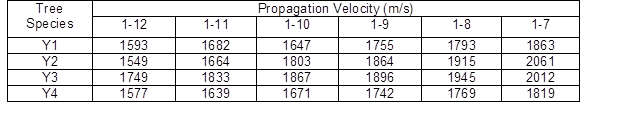Analysis of the Propagation Velocity of Stress Wave in Different Longitudinal Section Angles

When the orientation angle was 0, the stress wave propagation velocities of four kinds of healthy log samples in different longitudinal section angles between No.1- No.12 sensors were measured. The results are shown in Table 3.

Table 3. Stress Wave Propagation Velocity in Different Longitudinal Section Angles of Healthy Y1-Y4 Logs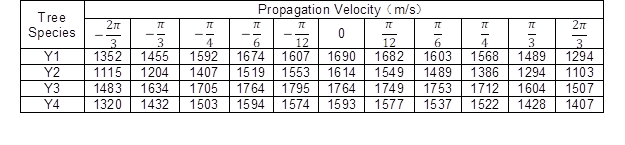The data in Table 3 were plotted as scatter points and fitted with curves, and the corresponding fitting equation was given, as shown in Fig. 7. The longitudinal section angle β is represented by abscissa x, the corresponding stress wave propagation velocity v is represented by longitudinal coordinate y, and the trend of stress wave propagation velocity in four samples in different longitudinal section angles are represented by four graphs, that is, the stress wave propagation velocity decreases with the increase of longitudinal section angles and the radial propagation velocity is the largest. The overall trend is a parabola downward from the opening, which is symmetrical about 0. The one-dimensional quadratic function relation y = ax2 + b + c is satisfied by analysis,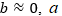depending on the physical and mechanical parameters of the sample (elastic modulus, density and moisture content, etc.), in accordance with the mathematical model of the theoretical model (Eq. 3), and the correlation coefficients R2 are above 0.92, the significance P is less than 0.01, and the theoretical and experimental mathematical models have a significant goodness of fit.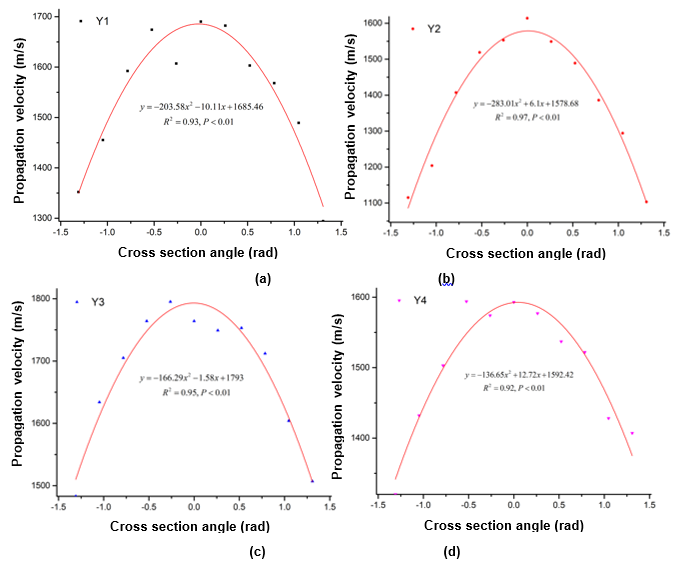Fig. 7. Fitting curve of stress wave propagation velocity in different longitudinal section angles of healthy Y1-Y4 logs

Analysis of the Propagation Velocity of Stress Wave in Longitudinal Section with Different Direction Angles

The larch logs were selected as the test sample, and the stress wave propagation velocities of longitudinal sections with different directions were measured by experiments (Fig. 3(C)). The cftool toolbox in Matlab software drawing function was used, combined with the mathematical model (z=Ax2-By2+Cx2y2-Dx2y4+E) of Eq. 4 to realize the three-dimensional spatial diagram drawing, as shown in Fig. 8. The x coordinate represents the direction angle (downward is positive, upward is negative), the y coordinate represents the intersection angle (clockwise is negative, counterclockwise is positive), and the z coordinate indicates the stress wave velocity. The three-dimensional surface map shows that the interaction between the direction angle and longitudinal section angle have a great influence on the propagation of the stress wave, which the propagation velocity of the stress wave propagates symmetrically with the direction angle and the longitudinal section angle.